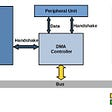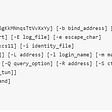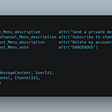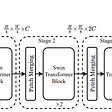# How to Implement an Efficient LayerNorm CUDA Kernel — OneFlow Performance Optimization

Written by Ran Guo, Chi Yao, Zekang Zheng, Juncheng Liu; Translated by Xiaozhen Liu, Hengrui Zhang

In a previous article, we discussed OneFlow’s techniques for optimizing the Softmax CUDA Kernel: How to implement an efficient Softmax CUDA kernel — — OneFlow Performance Optimization. The performance of the OneFlow-optimized Softmax greatly exceeds that of the Softmax of CuDNN, and OneFlow also fully optimizes `half` types that many frameworks do not take into account.

Here, we share OneFlow’s approach for optimizing the performance of another important operator, `LayerNorm`.

# Performance of OneFlow-optimized LayerNorm

The performance of OneFlow-optimized LayerNorm was tested compared with that of the LayerNorm of NVIDIA Apex, and of PyTorch respectively. The test results shows that OneFlow-optimized LayerNorm has distinct advantages.

## Comparison with the LayerNorm of NVIDIA Apex

NVIDIA Apex implements an efficient fused LayerNorm Kernel to extend PyTorch operators. We tested the OneFlow-optimized LayerNorm Kernel against the LayerNorm Kernel of NVIDIA Apex with the following results:

The horizontal axis is `num_cols` and the vertical axis is the time required for executing Kernel (lower is better):

Convert the time into access bandwidth and the result is as follows: The vertical axis is the effective bandwidth reached by the Kernel (the higher the better):

The test was conducted on the NVIDIA A100-PCIE-40GB GPU with the data type being `half`. `Shape = (49152， num_cols)`. We made the last dimensions dynamically vary, testing the LayerNorm Kernel for different sizes from 32 to 32768. The results show that the executing time of OneFlow-optimized Kernel is lower, and its effective access bandwidth is higher, making its performance better than Apex implementation.

## Comparison with the LayerNorm of PyTorch

PyTorch’s LayerNorm does not support the `half` type now, so we made a comparison based on the `float` type. It should be noted that the PyTorch' LayerNorm can be split into two CUDA Kernels (RowwiseMomentsCUDAKernel and LayerNormForwardCUDAKernel), so it seems to have poor performance.

The horizontal axis is `num_cols` and the vertical axis is the time required for executing Kernel (lower is better):

It can be seen from the above graphs that OneFlow’s LayerNorm has the best performance.

# OneFlow’s Approach for Optimizing LayerNorm

LayerNorm is one of the common operations for language models, and the efficiency of its CUDA Kernel will affect the final training speed of many networks. The Approach for Optimizing Softmax CUDA Kernel also applies to LayerNorm and the data of LayerNorm is also in the form of `(num_rows, num_cols)`. You can perform a Reduce operation on the elements of each row to calculate the mean and the variance. Therefore, we use the same optimization approach as Softmax to optimize the LayerNorm operation, and this paper introduces the forward algorithm for LayerNorm as an example.

## Forward Algorithm for LayerNorm

In PyTorch, for example, the LayerNorm interface is:

The `input` is in the shape of `[∗, normalized_shape, normalized_shape, …,normalized_shape[−1]]`.

The first parameter `normalized_shape` can only be the last several dimensions of `x_shape`. For example, if `x_shape` is `(N, C, H, W)`, `normalized_shape` can be `(W)`, `(H, W)`, `(C, H, W)` or `(N, C, H, W)`. Calculate the mean and variance of input `x` in dimension `normalized_shape`.

The third parameter `elementwise_affine` is to choose whether to transform the result of normalize (multiply the result of normalize by `gamma` and add `beta`). If `elementwise_affine=True`, two parameters `gamma` and `beta` are added, and their shapes are `normalized_shape`.

For example, if the input `x` is `(N, C, H, W)` and the `normalized_shape` is `(H, W)`, it can be understood that the input `x` is `(N*C, H*W)`, namely each of the `N*C` rows has `H*W` elements. Get the mean and variance of the elements in each row to obtain `N*C` numbers of `mean` and `inv_variance`, and then calculate the input according to the following LayerNorm formula to get `y`. If `elementwise_affine=True`, then `H*W` numbers of `gamma` and `beta` are performing transformation for `H*W` numbers of elements in each row.

## Algorithms for Calculating Variance for LayerNorm

The common algorithms for calculating variance are two-pass algorithm, Naïve algorithm, and Welford’s online algorithm. This article extracts some key formulas and conclusions, detailed introduction and derivation can be referred to Wiki: Algorithms for calculating variance and GiantPandaCV: Using Welford’s online algorithm to update the variance for LN.

• Two-pass algorithm

The formula is:

“Two-pass” means this algorithm needs to iterate through the data twice. First computes the sample mean by accumulation, and then then computes the sum of the squares of the differences from the mean. This algorithm is numerically stable if `n` is small.

• Naïve algorithm

The formula is:

This is a single-pass algorithm. To calculate the variance, you only need to iterate through the data once to accumulate the square of `x` and accumulate `x`, and then calculate the variance based on the above formula. This method only needs to iterate through the data once, which is easier to achieve good performance than the two-pass algorithm. But it is not recommended in practice because the result of SumSquare and (Sum×Sum)/n might be very close to each other, which may lead to loss of precision, as described in the Wiki reference link above.

• Welford’s online algorithm

The formula is:

Welford’s online algorithm is also a single-pass algorithm and numerically stable. Therefore, many frameworks adopts to this algorithm. The codes in this article also adopt it.

## OneFlow’s Approach for Deep Optimization of LayerNorm CUDA Kernel

Like Softmax, LayerNorm is also optimized using a segmentation function in OneFlow: for different `num_cols` ranges, different implementations are chosen in order to achieve a high effective bandwidth in all cases.

In each implementation, a common optimization is adopted: vectorized memory access. On NVIDIA, the blog Increase Performance with Vectorized Memory Access mentioned that you can use vector loads and stores to increase the performance of CUDA Kernel. This is because many CUDA kernels are bandwidth bound, and vectorized memory access helps decrease the number of executed instructions, reduce the latency and increase bandwidth utilization.

Theoretically, during the computation of the LayerNorm, the input `x` needs to be read twice: the first time for calculating the mean and variance and the second time for the later computation after the mean and variance are obtained. But the access to Global Memory is expensive, so if the input `x` is stored first and not read, the performance can be improved. In GPU, input `x` can be stored in registers or Shared memory, but the resources are limited. If `num_cols` is too large, it will exceed the resource limit. So we use different implementations for different `num_cols`, which are described below.

When `num_cols<=1024`

When `num_cols<=1024`, a warp processes one or two rows of computation and stores input `x` in a register.

32 threads executing in parallel on the hardware are called a warp, and 32 threads of the same warp execute the same instruction. A warp is the basic unit of GPU scheduling and execution. The correspondence between thread blocks and elements is shown in the figure above. The threads for each warp process a row of elements, and each block has `block_ size / warp_ Size` warps, and processes `block_ size / warp_ Size` rows of element.

The specific processing flow is shown in the following figure. There are `num_cols` elements in each row, and each warp processes one row, so each thread needs to process `num_cols / warp_size` elements. Each thread reads the elements and stores them in the registers. After calculating the mean and variance using the Welford's online algorithm, all threads in warp perform a WelfordwarpAllReduce, so that each thread gets the correct mean and variance for later calculations.

WelfordwarpAllReduce is performed by WelfordwarpReduce and Broadcast. WelfordwarpReduce is supported by warp shuffle function `__shfl_down_sync` and Broadcast is supported by `__shfl_sync`. The code is as follows:

There is a template parameter `thread_group_width` where when `num_cols > pack_size * WarpSize`, `thread_group_width` is `WarpSize`. When `num_cols` is too small, i.e., `num_cols <= pack_size * WarpSize`, the threads within a Warp are not all valid values, so a smaller `thread_group_width` is used. The value can be 16, 8, 4, 2, or 1, depending on `num_cols`. At this time, each thread processes two rows to increase the degree of parallelism.

In addition, we use vectorized memory access to optimize the read and write operations of input and output. When the value of input and output meets a certain condition, pack `pack_size` elements into a larger data type for read-in. The following graph is an example when `pack_size=2`. In this case, Each thread reads elements with a larger data type, which can make better use of the video memory bandwidth.

When we pack `pack_size` elements into a larger data type, `x` is still needed for calculation. Therefore, we define a `Pack` type with a `union` structure. Storage is used to read and write from global memory. When doing calculations, use `elem[i]` to take each element to calculate. The `Pack` type is defined as follows:

The code of LayerNormWarpImpl Kernel is as follows:

The meanings of the template parameters in the implementation of LayerNormWarpImpl are as follows:

• `LOAD` and `STORE` represent input and output respectively. Use `load.template load<pack_size>(ptr, row_id, col_id);` and `store.template store<pack_size>(ptr, row_id, col_id);` to read and write. There are two advantages of using `LOAD` and `STORE`: first, you can only consider `ComputeType` rather than the specific data type `T` in CUDA Kernel; second, just a few more lines of code can support LayerNorm and other Kernel Fuse, and reduce bandwidth demands to improve overall performance.
• `ComputeType` means the type of computing. `pack_size` means the number of pack elements for vectorized memory access operation. Packing several elements for read and write can improve bandwidth utilization.
• `cols_per_thread` represents the number of elements processed by each thread.
• `thread_group_width` represents the width of the thread group that processes elements. When `cols > pack_size * warp_size`, `thread_group_width` is `warp_size`, which is 32. When `cols < pack_size * warp_size`, the elements of each row are processed by 1/2 warp or 1/4 warp according to the size of cols. A smaller `thread_group_width` means a lower number of rounds that WarpAllReduce needs to execute.
• `rows_per_access` represents the number of rows processed by each thread_group at a time. When `cols` is small and `thread_group_width` is less than `warp_size`, if `rows` is divisible by 2, each thread processes 2 rows to increase the parallelism of instructions to improve performance.
• `padding` represents whether padding is currently done. If `cols` is not an integer multiple of `warp_size`, it will be converted into the nearest integer multiple with padding.

When `num_cols > 1024`

When `num_cols > 1024`, process a row in block unit, and use Shared Memory to store input data.

For `num_cols > 1024`, each block processes a row of elements and stores the input `x` in Shared Memory.

The specific processing flow is shown in the following graph. There are `num_cols` elements in each row, and each graph processes one row, so each thread needs to process `num_cols / block_size` elements. Each thread reads the elements and stores them in the shared memory. After calculating the mean and variance using the Welford's online algorithm, all threads in block perform a WelfordblockAllReduce, so that each thread gets the correct mean and variance for later calculations.

WelfordBlockAllReduce is implemented with the WelfordWarpReduce operation. The specific logic is that there are at most 32 warps in a Block. First, WelfordWarpReduce is executed once for all warps. Then, the first thread in each warp, namely the thread with `lane_id=0`, gets the result of WelfordWarpReduce opertation. Then the result of the first thread of each warp is copied to a Shared Memory buffer, and WelfordWarpReduce is executed with the 32 threads of the first warp. At this time, in the first warp the thread with `lane_id=0` is the result after the reduce of all threads in the block. At last, with Shared Memory, the result is broadcast to all threads in the block, which completes the operation of WelfordBlockAllReduce.

Note that Shared Memory resources on the GPU are also limited. When `num_cols` exceeds a certain range, the Shared Memory that needs to be occupied may exceed the maximum limit, and the Kernel cannot be launched.

Therefore, we use the `cudaOccupancyMaxActiveBlocksPerMultiprocessor` function to determine whether the Kernel can be successfully launched under the current hardware resource conditions, and only use this scheme when the return value is greater than 0.

In addition, because the threads in the block need to be synchronized, when a block scheduled for execution in the SM reaches the synchronization point, the executable Warp in the SM gradually decreases. If there is only one block that is executed, the Warp that can be executed simultaneously in the SM will gradually decrease to 0 at this time, leading to idle computing resources and waste. If other blocks are executing at the same time, there are still other blocks that can be executed when a block reaches the synchronization point.

The smaller `block_size` is, the more blocks SM can schedule at the same time. So in this case, the smaller the `block_size`, the better. But when `block_size` is increased and the number of blocks that SM can schedule at the same time remains the same, `block_size` should be the larger the better, because the larger the block, the better the degree of parallelism. Therefore, in the code, when consider `block_size`, `cudaOccupancyMaxActiveBlocksPerMultiprocessor` should be calculated for different `block_size`. If the results are the same, use a larger `block_size`.

The code of LayerNormBlockSMemImpl Kernel is as follows:

When num_cols is Relatively Large and Shared Memory is Not Used

When `num_cols` is large and Shared Memory cannot successfully launch Kernel under current hardware conditions, try this: a block processes a row of elements without using Shared Memory, and repeatedly reads input `x`.

In this method, the relationship between threads and elements is the same as that in the second scenario mentioned before. The only difference is that the second scenario stores the input `x` in Shared Memory but this method does not store `x`. In each calculation, it reads in `x` from Global Memory. Although this method needs to read an extra copy of `x`, in actual execution, part of the input can be cached by the Cache, which will not waste much time. It should be noted that in this method, the larger the `block_size`, the smaller the number of blocks that can be executed in parallel in the SM, the less demand for the Cache, and the more opportunities to hit the Cache. Therefore, we use the larger `block_size`.

The code of LayerNormBlockUncachedImpl is as follows:

Related articles：

Also, welcome to join our Discord group to discuss and ask OneFlow related questions, and connect with OneFlow contributors and users all around the world.

--

--

--

## More from OneFlow

OneFlow is a performance-centered and open-source deep learning framework.

Love podcasts or audiobooks? Learn on the go with our new app.

## What is DMA and how does it work?## Insert ROW-WISE Values into DaTaFrAmE using PYTHON## Live Streaming Video Chat App using cv2 module of Python..## Importance of Dataset Management command in SSD## What are SOLID Principles## Create and deploy an AWS Lambda function with AWS CloudFormation## Compile-Time Introspection of Sum Types in Pure C99## OneFlow

OneFlow is a performance-centered and open-source deep learning framework.

## OneFlow’s Optimization of CUDA Elementwise Template Library: Practical, Efficient, and Extensible## Overview — Video Swin Transformer## SimCLR — STL10 Implementation## Swin Transformer 🚀: Hierarchical Vision Transformer using Shifted Window — Part I